How to use JMP and MATLAB together

Extensibility is one of the hallmarks of JMP. In JMP 11, you can integrate JMP with MATLAB. From JMP Scripting Language (JSL), you can send data from JMP to MATLAB, execute MATLAB functions and return results from MATLAB to JMP for further analysis and visualization.

MATLAB, a software solution from MathWorks, is a matrix programming language and interactive programming environment for numerical computation, data analysis, visualization, and building custom engineering models and applications. It includes a rich set of add-on modules, known as toolboxes, which give MATLAB users access to functions to perform specialized modeling and design work as needed.

The MATLAB interface in JMP provides access to MATLAB through JSL with a set of functions. The basic execution model is to first initialize a MATLAB connection, perform the required MATLAB operations and then terminate the MATLAB connection. The usage paradigm is very similar to the R interface in JMP.

In this post, I wanted to briefly highlight how to get the interface up and running on your JMP 11 installation, and present some basic examples of how to use the interface. For a deeper dive into the specifics of the interface, I suggest downloading the white paper that I co-authored with John Salmon, PhD, a research engineer in the Aerospace Systems Design Laboratory at the Georgia Institute of Technology.

First things, first: Let’s get the installation instructions out of the way. Detailed instructions are available in our online help, but here is a quick-start guide.

• MATLAB must be installed on the same computer as JMP, and JMP is not distributed with a copy of MATLAB.
• Because JMP is supported as both a 32-bit and 64-bit Windows application, you must install the corresponding 32-bit or 64-bit version of MATLAB.
• JMP delays loading MATLAB until a JSL script requires access to it. When JMP needs to load MATLAB, it is located based on the current search path using a PathVar variable. This should be set using the platform preferences of the MATLAB interface.
• Setting the MATLABROOT to the path of the MATLAB installation on your computer will make sure JMP knows where to find MATLAB (as shown in the figure below).
•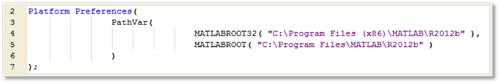After the path has been set in the platform preferences, you can perform a quick check to make sure that your MATLAB connection is working by running a MATLAB Init(); function. If the connection has been successful, the function will return 0 to the log. If the operation was not successful, you will get an error message.

So let’s get right into an example of how the interface works. This example shows each stage of the interface workflow, so it serves as a good prototype of more complex scripts. The first step is to initialize the MATLAB connection with a MATLAB Init(); call. Then, we use a MATLAB Submit(); function to execute some MATLAB code in MATLAB. In this case, we are running the “magic” function with an input parameter of 3 and storing the results into a variable named m. In order to bring the m matrix into the JMP variable space for further use of the results in JMP, we run the MATLAB Get(); function, which takes the data stored in m and assigns it to a JMP variable, which we have named: magicMat. To see the results of our small program, we write the contents of magicMat to the log and bring the data into a JMP data table as shown in figure below. And finally, we end our MATLAB session with a MATLAB Term(); command.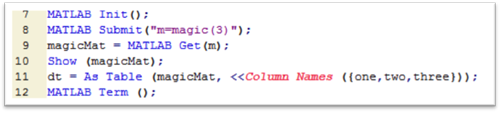The benefit here is that the data from our MATLAB program is now in a JMP data table and ready to be visualized, modeled or explored without any further MATLAB coding. You also gain the interactivity of JMP for exploring MATLAB model output and can use JMP tools for data visualization (e.g., via Graph Builder, Control Chart Builder, Distribution), data analysis (Fit Model, Partition, Screening, etc.) as well as additional data cleanup and summarization (tools in the Tables menu, Tabulate, etc.).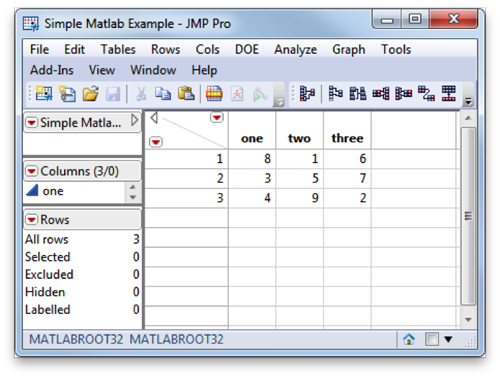Now that we have done something basic, let’s have a little bit more fun. One of my hobbies outside of work is speaker design and building. Below is a picture of my current design in progress. We will see how I can use MATLAB and JMP to help me in my design and tuning of these speakers. One of the great things that I have added to my setup in the past year is a MiniDSP. The MiniDSP is an amazing little device that has made rapid prototyping of speakers and crossovers much easier, and it has also saved me the hassle and expense of having to buy passive crossover components.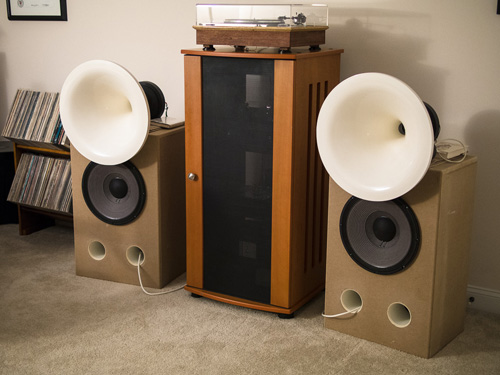The MiniDSP lets me do a number of things: time alignment, low- and high-pass crossover filters and room correction. How do I know what I need to do to make my speakers sound best in my room? I create what’s known as an impulse response (IR) by playing a swept sine wave measurement signal through the speakers and then recording the output with a microphone. Subtracting the dry signal from the wet, measured signal creates the IR. What is left is data set that describes how sound decays in my room. But what I need to know really is not really that data that is in the time domain, but rather the frequency response of my speakers. Fortunately, this is easy to analyze given the time-domain data by using a Fast Fourier Transform (FFT) algorithm.

The example that I am going to show you is a measurement I made when I was trying to verify how close the turning of my bass reflex ports were to the model I built of the cabinet. For this, I placed the microphone close to the speaker in between both ports on my left speaker. I don’t have to worry about room reflections for this because the direct sound from the ports is so much greater in amplitude than the reflected sound. To look at the broadband response from the speakers, I am going to have to employ a different method (which involves bringing the speakers outside and putting them up on a pedestal and then windowing out reflections – but that will have to wait until it is a little bit warmer).

So here is the time-domain data of my measurement: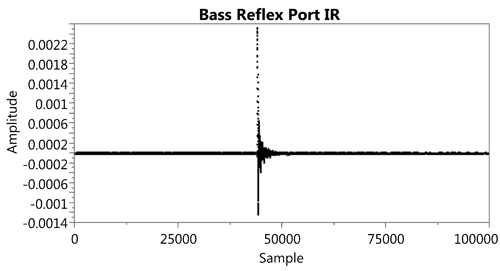What I really want to do is convert this the frequency domain so that we can plot the response and answer our question: Is the port resonating where it should be (about 55 Hz)?

For this analysis, I want to take the data from JMP, send it to MATLAB, execute a function that I’ve written to perform the FFT and return the resulting vector back to a new data table with the properly labeled frequency bins (by telling my MATLAB function what my sample rate is).

To automate this analysis in the future for new measurements, I’ve turned this analysis into a new JMP application, which can then be packaged as an add-in and added to my JMP menu. It’s a simple dialog that asks for the column, which has the amplitude data.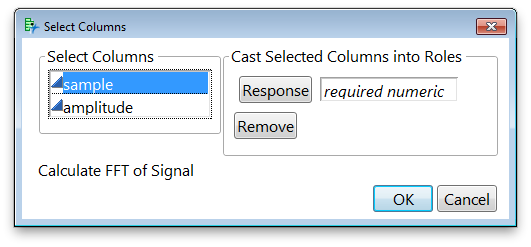When I press OK, it will execute the JSL that will round-trip my data to MATLAB, perform the FFT and return the results of the analysis back to a data table. The code actually required to run the MATLAB script is quite simple. In line 26, I send the variable yvec, which corresponds to the response role that the user has selected in the application to the MATLAB variable space. Then, I submit the MATLAB code required to run my FFT function. In my case, I know all of my IRs are sampled at 44.1 kHz, so I have hard-coded that into the input list for my calcFFT function. If the sample rate was varying, I could add a text input field to my application and then pass the selection to my function call.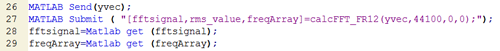My function returns two vectors that I am interested in, the fftsignal and the freqArray. I can then pull those two variables into the JMP variable space with the MATLAB Get(); function and create a table from the results.

After I have my results, I can use Graph Builder to generate my frequency response graph: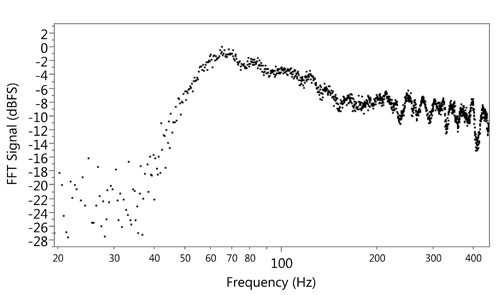I’ve focused on just the frequency response from 20 Hz – 400 Hz so I can see the port tuning behavior (which appears as a peak on the graph). It is a little bit higher than I expected based on my model, so I may need to add a little bit of a bass-boost to the low frequency channel in the MiniDSP in order to have the frequency response I need in my room.

I’ve just shown one example of where we can use JMP and MATLAB together to extend the capabilities of JMP. In the white paper, we go through several other examples, including using JMP to set up a design of experiments using the Custom Designer, run simulation experiments in MATLAB, and then return the results to JMP for analysis, factor profiling and assessing variable importance.

Have you used the JMP interface to MATLAB in JMP 11 yet? If you have, I would love to hear your story!

Article Labels

There are no labels assigned to this post.

Article Tags
Visitor

emmnuel wrote:

Can u share the complete script?Staff

Daniel Valente wrote:

Hello Emmanuel --

You can download the sample scripts from the White Paper: "JMP Extensibility Synergy with MATLAB" on our File Exchange.

Visitor

emmanuel wrote:

There are some errors in scripts, i also was seeing one of the videos and there are some typos.

First example have Colum name error, in the log. so i have to eliminate that part to:

MATLAB Init();

MATLAB Submit("m=magic(3)");

magicMat= MATLAB get(m);

show (magicMat);

dt = as table (magicMAt);

MATLAB Term( );

So it could work

The FFT si the part that i am interest, it should start wih MATLAB Init();

I currently have an error in the log.

"Undefined function 'calcFFT_FR12' for input arguments of type 'double'."

Can you please also send me the the data sample of 25,000?

So i can review it

Visitor

emmanuel wrote:

Sorry i just download the file, it seems there is the complete pack, thanksStaff

Daniel Valente wrote: